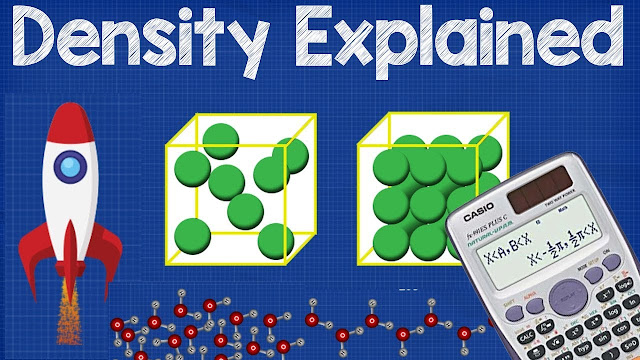What is Density? - Density Explained

# What is Density? - Density ExplainedWhat is Density? - Density Explained

The density (more precisely, the volumetric mass density; also known as specific mass), of a substance is its mass per unit volume. The symbol most often used for density is ρ (the lower case Greek letter rho), although the Latin letter D can also be used. Mathematically, density is defined as mass divided by volume.
where ρ is the density, m is the mass, and V is the volume. In some cases (for instance, in the United States oil and gas industry), density is loosely defined as its weight per unit volume, although this is scientifically inaccurate – this quantity is more specifically called specific weight.

For a pure substance the density has the same numerical value as its mass concentration. Different materials usually have different densities, and density may be relevant to buoyancy, purity and packaging. Osmium and iridium are the densest known elements at standard conditions for temperature and pressure.

To simplify comparisons of density across different systems of units, it is sometimes replaced by the dimensionless quantity "relative density" or "specific gravity", i.e. the ratio of the density of the material to that of a standard material, usually water. Thus a relative density less than one means that the substance floats in water.

The density of a material varies with temperature and pressure. This variation is typically small for solids and liquids but much greater for gases. Increasing the pressure on an object decreases the volume of the object and thus increases its density. Increasing the temperature of a substance (with a few exceptions) decreases its density by increasing its volume. In most materials, heating the bottom of a fluid results in convection of the heat from the bottom to the top, due to the decrease in the density of the heated fluid. This causes it to rise relative to more dense unheated material.

The reciprocal of the density of a substance is occasionally called its specific volume, a term sometimes used in thermodynamics. Density is an intensive property in that increasing the amount of a substance does not increase its density; rather it increases its mass.

What is density? in this video we explain in simple terms what density is, why it is important, how to calculate density in both imperial and metric units as well as worked examples to help you learn and apply your knowledge.Density is calculated by dividing the mass by the volume. Density explains why ice floats. If you freeze water it expands.

Related topics:

Continue Reading about the "What is Density? - Density Explained" on the next page below